# Introduction

In spatial predictive mapping, models are often applied to make predictions far beyond sampling locations (i.e. field observarions used to map a variable even on a global scale), where new locations might considerably differ in their environmental properties. However, areas in the predictor space without support of training data are problematic. The model has no knowledge about these environments and predictions for such areas have to be considered highly uncertain.

Here we implement the methodology described in Meyer&Pebesma (2020) to estimate the “area of applicability” (AOA) of spatial prediction models. The AOA is defined as the area where we enabled the model to learn about relationships based on the training data, and where the estimated cross-validation performance holds. To delineate the AOA, first an dissimilarity index (DI) is calculated that is based on distances to the training data in the multidimensional predictor variable space. To account for relevance of predictor variables responsible for prediction patterns we weight variables by the model-derived importance scores prior to distance calculation. The AOA is then derived by applying a threshold based on the DI observed in the training data using cross-validation.

This tutorial shows an example of how to estimate the area of applicability of spatial prediction models.

For further information see: Meyer, H., Pebesma, E. (2020): Predicting into unknown space? Estimating the area of applicability of spatial prediction models. [https://arxiv.org/abs/2005.07939]

### Getting started

``````library(CAST)
library(virtualspecies)
library(caret)
library(raster)
library(sp)
library(sf)
library(viridis)
library(latticeExtra)
library(gridExtra)``````

# Example 1: Using simulated data

## Get data

### Generate Predictors

As predictor variables, a set of bioclimatic variables are used (https://www.worldclim.org/). For this tutorial, they have been originally downloaded using the getData function from the raster package but cropped to an area in central Europe. The cropped data are provided in the CAST package.

``````predictors <- stack(system.file("extdata","bioclim.grd",package="CAST"))
spplot(stretch(predictors,0,1),col.regions=viridis(100))``````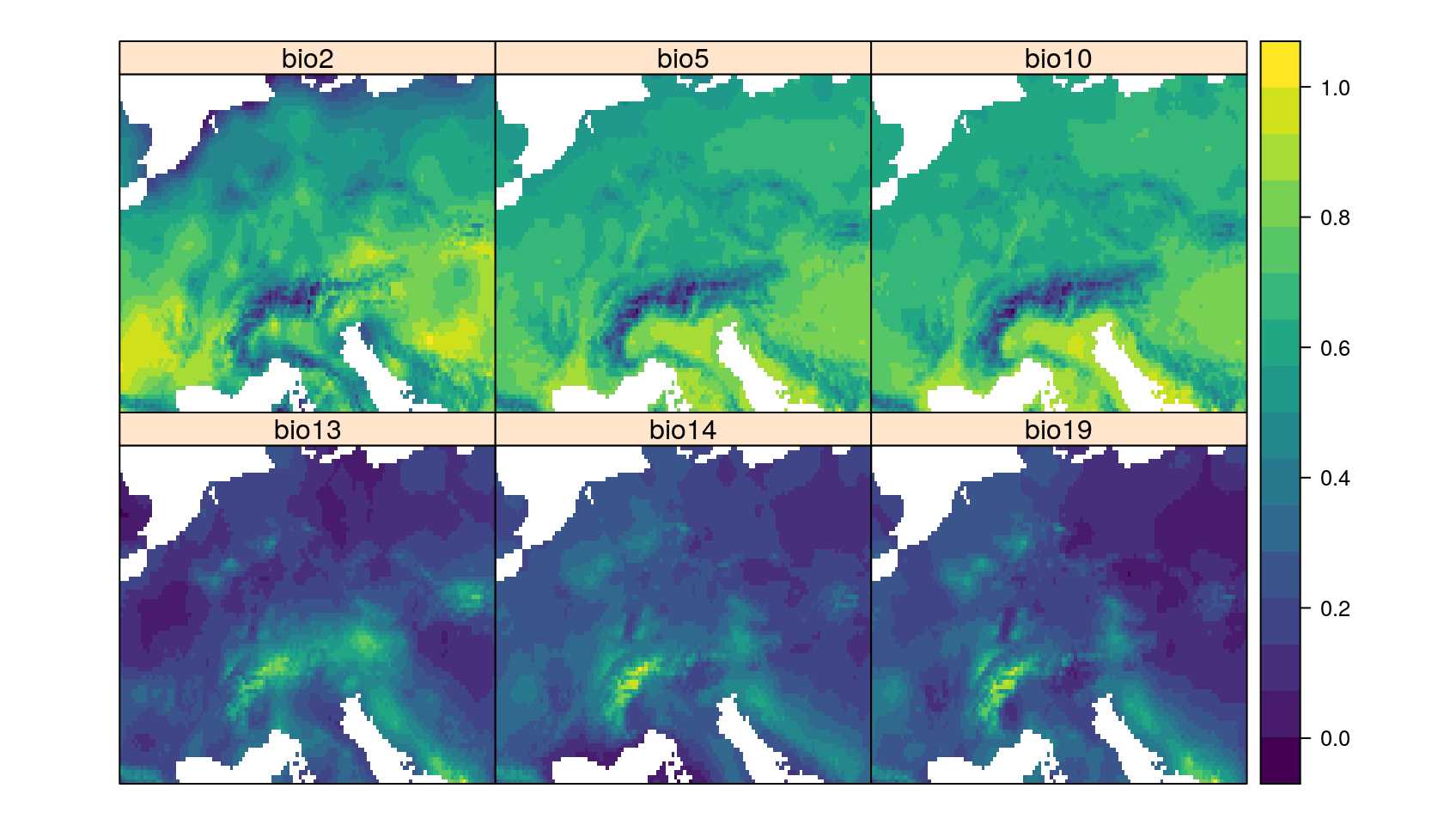### Generate Response

To be able to test the reliability of the method, we’re using a simulated prediction task from the virtualspecies package. Therefore, a virtual response variable is simulated from the bioclimatic variables. See Leroy et al. 2016 for further information on this methodology.

``````response <- generateSpFromPCA(predictors,
means = c(3,1),sds = c(2,2), plot=F)\$suitab.raster``````

### Simulate sampling locations

To simulate a typical prediction task, field sampling locations are randomly selected. Here, we randomly select 20 points. Note that this is a very small data set, but used here to avoid long computation times.

``````mask <- predictors[]
set.seed(15)
spplot(response,col.regions=viridis(100),
sp.layout=list("sp.points", samplepoints, col = "red", first = FALSE, cex=2))``````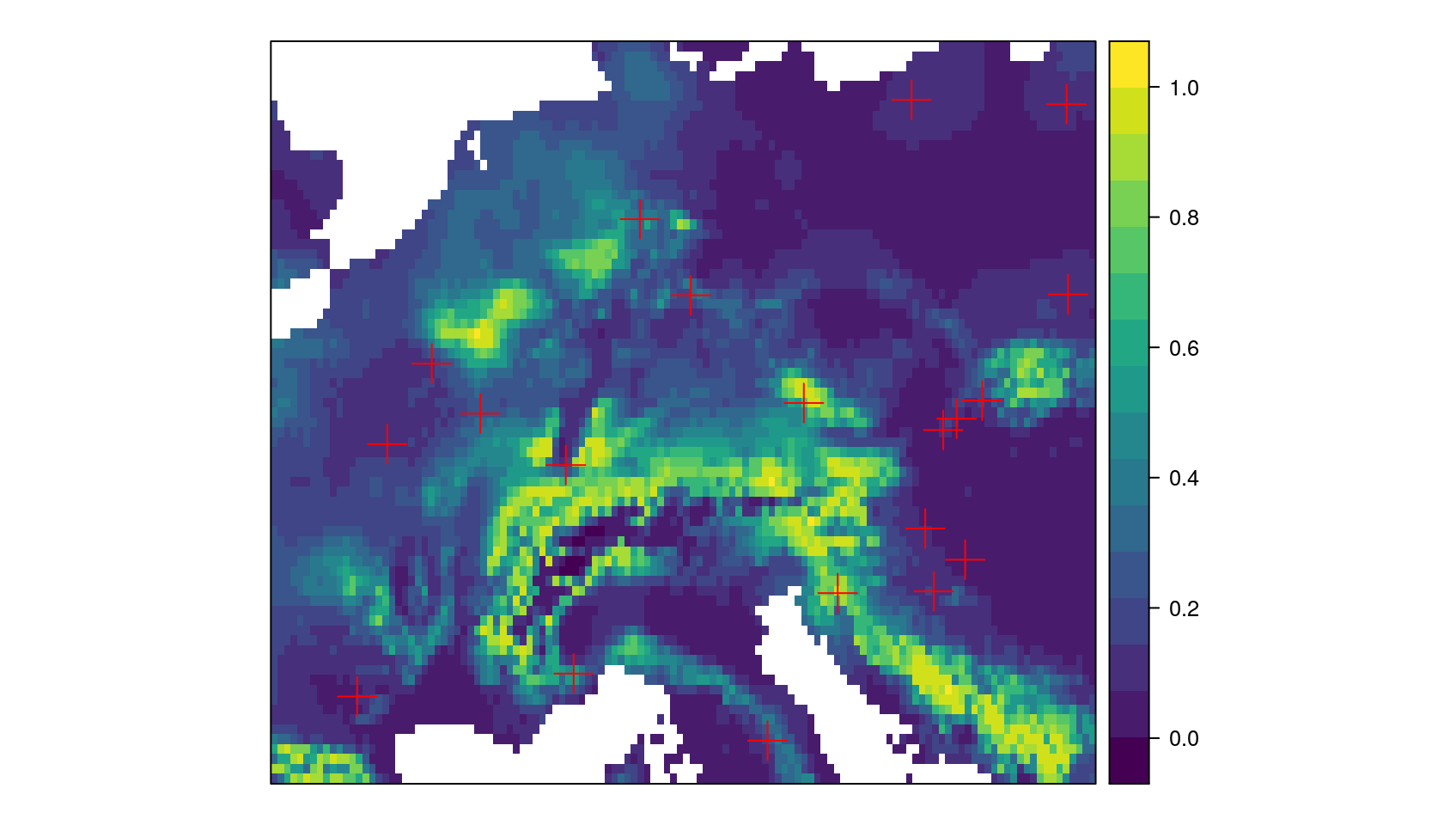## Model training

Next, a machine learning algorithm will be applied to learn the relationships between predictors and response.

### Prepare data

Therefore, predictors and response are extracted for the sampling locations.

``````trainDat <- extract(predictors,samplepoints,df=TRUE)
trainDat\$response <- extract (response,samplepoints)
trainDat <- trainDat[complete.cases(trainDat),]``````

### Train the model

Random Forest is applied here as machine learning algorithm (others can be used as well, as long as variable importance is returned). The model is validated by cross-validation to estimate the prediction error.

``````set.seed(10)
model <- train(trainDat[,names(predictors)],
trainDat\$response,
method="rf",
importance=TRUE,
trControl = trainControl(method="cv"))
print(model)``````
``````## Random Forest
##
## 20 samples
##  6 predictor
##
## No pre-processing
## Resampling: Cross-Validated (10 fold)
## Summary of sample sizes: 18, 18, 18, 18, 18, 18, ...
## Resampling results across tuning parameters:
##
##   mtry  RMSE       Rsquared  MAE
##   2     0.1103267  1         0.0962007
##   4     0.1200531  1         0.1025987
##   6     0.1187460  1         0.1014125
##
## RMSE was used to select the optimal model using the smallest value.
## The final value used for the model was mtry = 2.``````

### Variable importance

The estimation of the AOA will require the importance of the individual predictor variables.

``plot(varImp(model,scale = F),col="black")``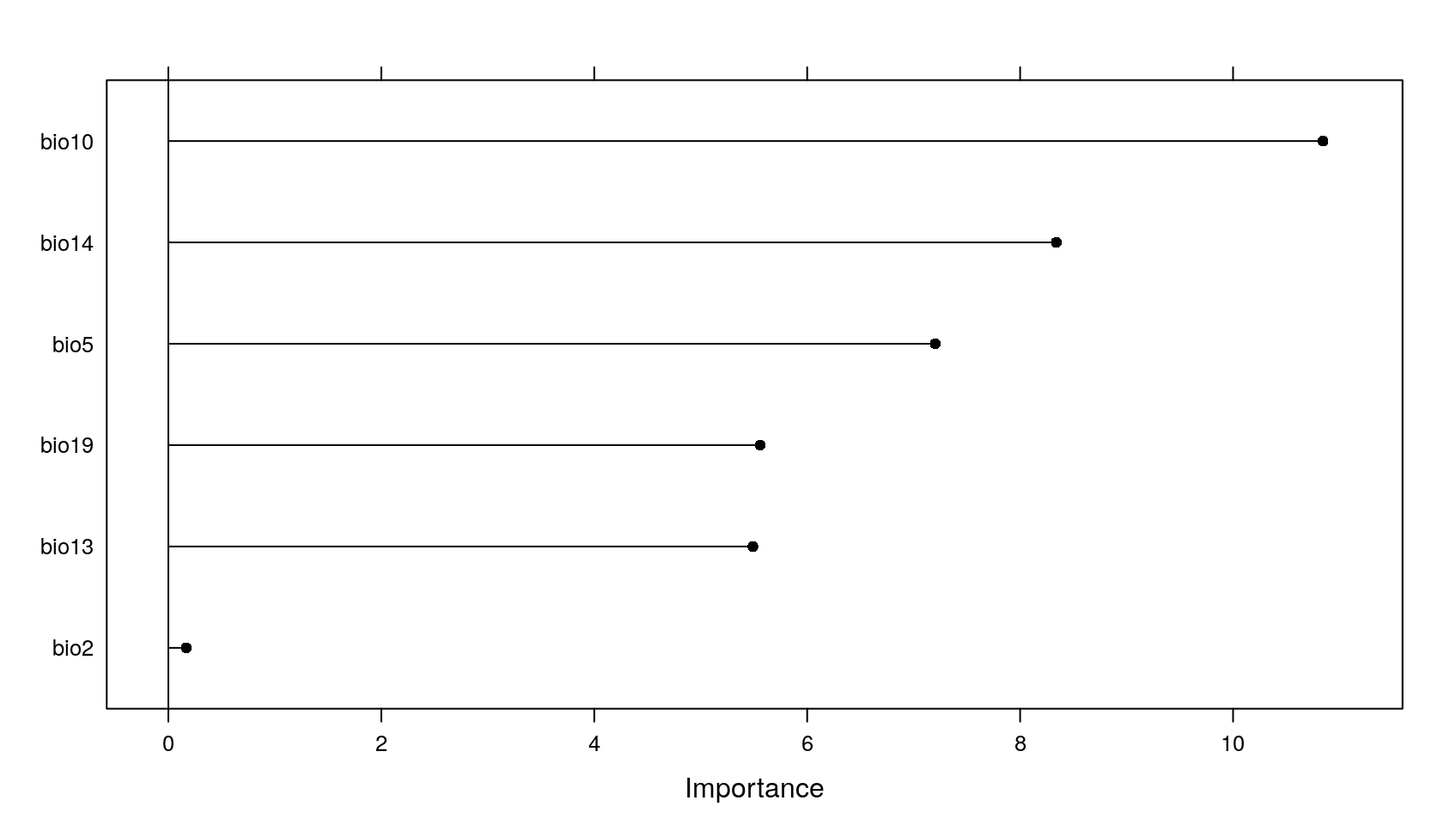### Predict and calculate error

The trainined model is then used to make predictions for the entire area of interest. Since a simulated area-wide response is used, it’s possible in this tutorial to compare the predictions with the true reference.

``````prediction <- predict(predictors,model)
truediff <- abs(prediction-response)
spplot(stack(prediction,response),main=c("prediction","reference"))``````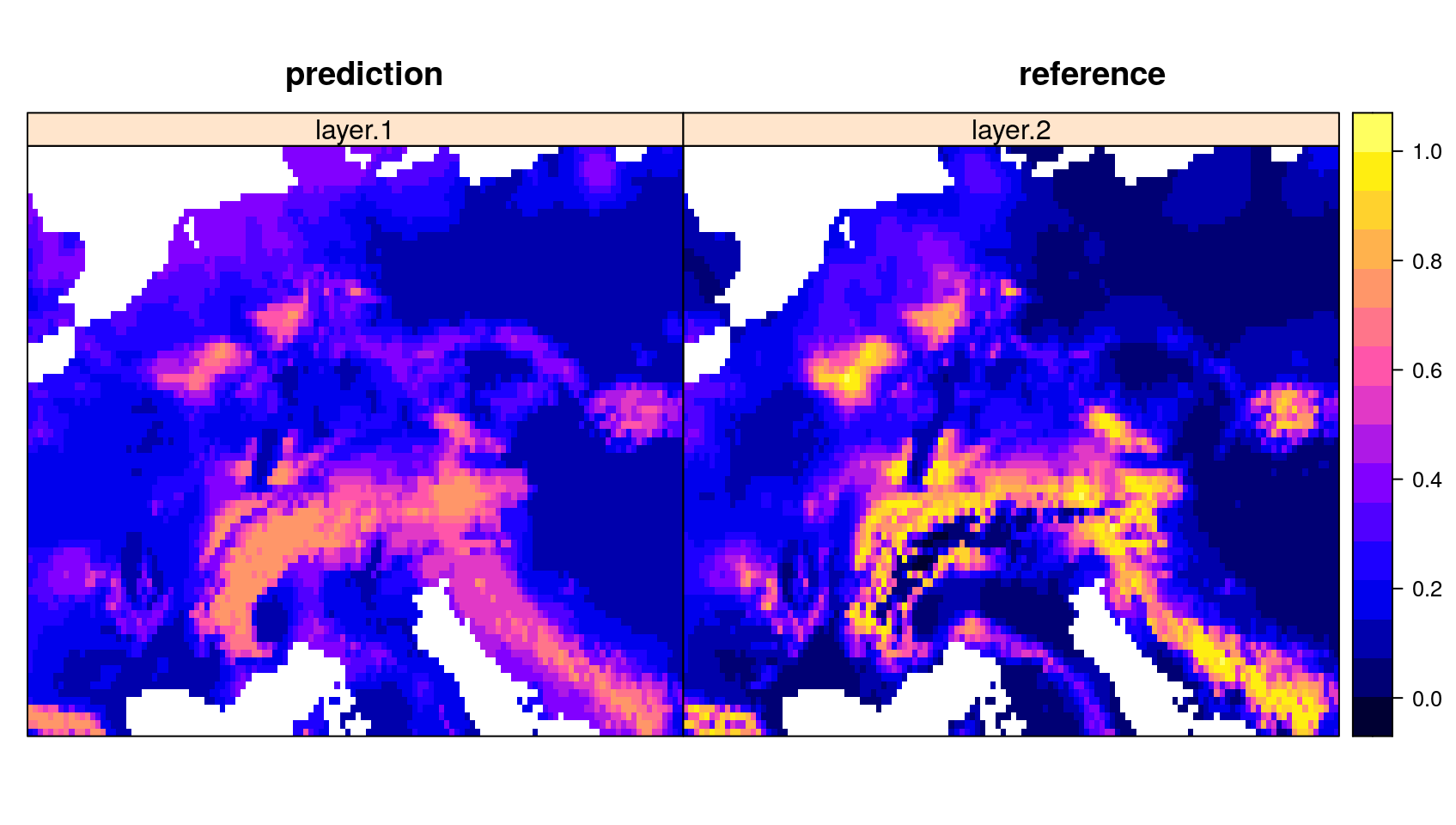## AOA Calculation

The visualization above shows the predictions made by the model. In the next step, the DI and AOA will be calculated.

The AOA calculation takes the model as input to extract the importance of the predictors, used as weights in multidimensional distance calculation. Note that the AOA can also be calculated without a trained model (i.e. using training data and new data only). In this case all predicor variables are trated equally important (unless weights are given in form of a table).

``````AOA <- aoa(predictors, model)
attributes(AOA)\$aoa_stats``````
``````## \$Mean_train
##  21.70346
##
## \$threshold_stats
##       25%       50%       75%       90%       95%       99%      100%
## 0.2542871 0.3018769 0.4296601 0.4942615 0.5989617 0.8104758 0.8633543
##
## \$threshold
##  0.5850463
##
## \$lower_threshold
##  0.1000228``````

The output of the aoa function are two raster data: The first is the DI that is the normalized and weighted minimum distance to a nearest training data point divided by the average distance within the training data. The AOA is derived from the DI by using a threshold. The threshold is the (outlier-removed) maximum DI observed in the training data where the DI of the training data is calculated by considering the cross-validation folds. The used threshold is returned in the AOA statistics.

``````grid.arrange(
spplot(truediff,col.regions=viridis(100),main="true prediction error"),
spplot(AOA\$DI,col.regions=viridis(100),main="DI"),
spplot(prediction, col.regions=viridis(100),main="prediction for AOA")+ spplot(AOA\$AOA,col.regions=c("grey","transparent")), ncol=3)``````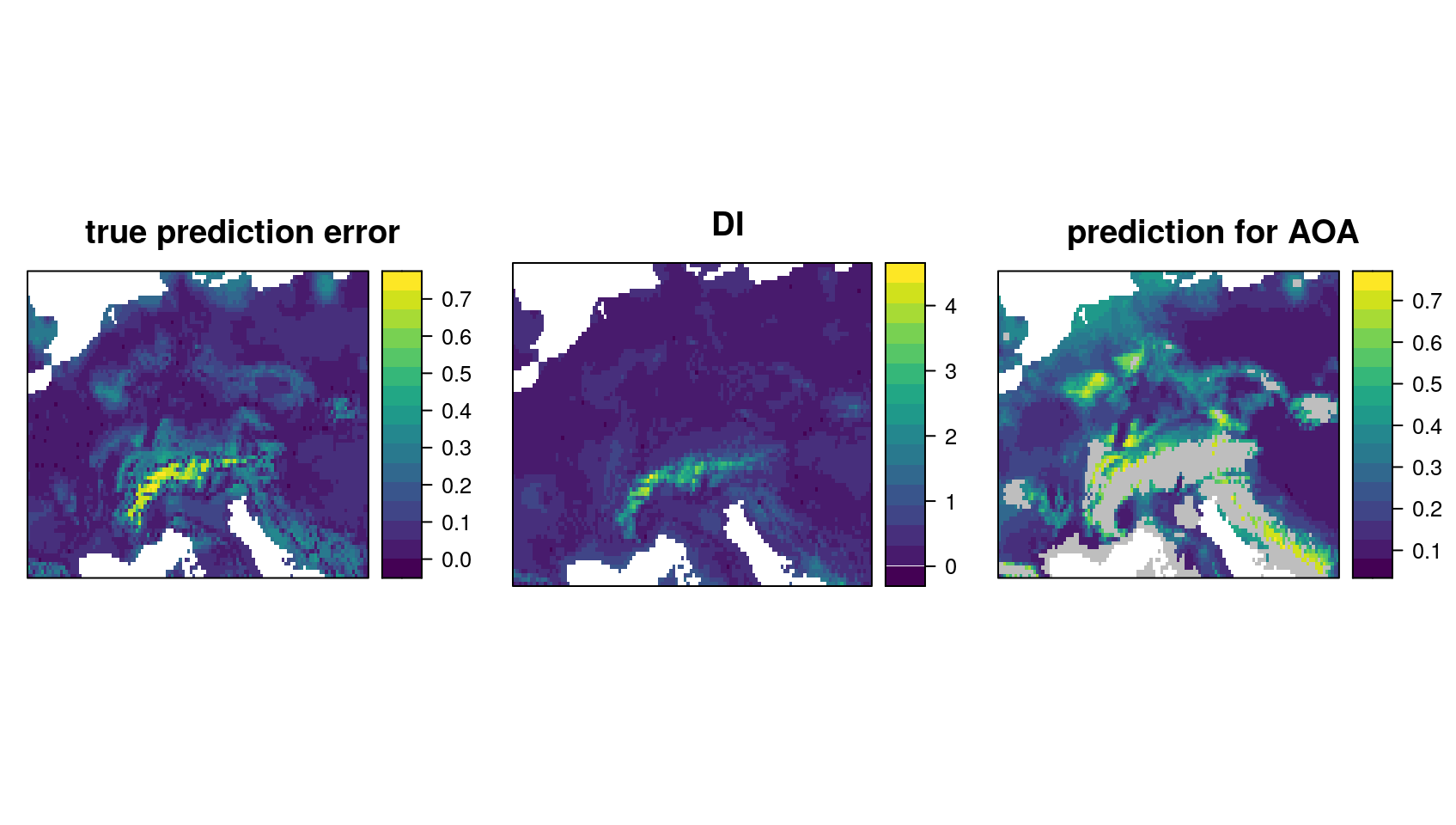The patterns in the DI are in general agreement with the true prediction error. Very high values are present in the Alps, as they have not been covered by training data but feature very distinct environmental conditions. Since the DI values for these areas are above the threshold, we regard this area as outside the AOA.

## AOA for spatially clustered data?

The example above had randomly distributed training samples. However, sampling locations might also be highly clustered in space. In this case, the random cross-validation is not meaningful (see e.g. Meyer et al. 2018, Meyer et al. 2019, Valavi et al. 2019, Roberts et al. 2018, Pohjankukka et al. 2017, Brenning 2012)

Also the threshold for the AOA is not reliable, because it is based in distance to a nearest data point within the training data (which is usually very small when data are clustered). Instead, cross-validation should be based on a leave-cluster-out approach, and the AOA estimation based on distances to a nearest data point not located in the same spatial cluster.

To show how this looks like, we use 15 spatial locations and simulate 5 data points around each location.

``````samplepoints <- csample(mask,75,15,maxdist=0.20,seed=15)
spplot(response,col.regions=viridis(100),
sp.layout=list("sp.points", samplepoints, col = "red", first = FALSE, cex=2))``````